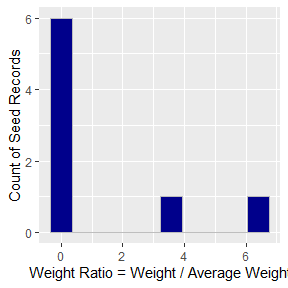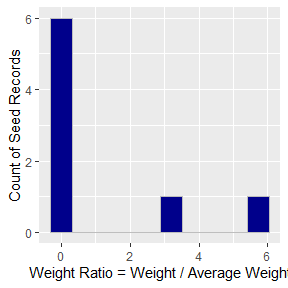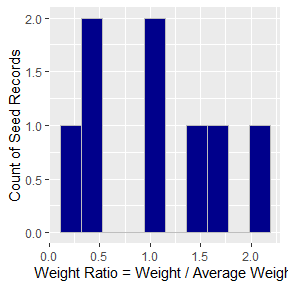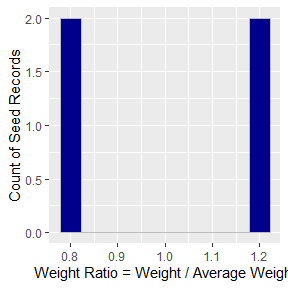suppressPackageStartupMessages({
library(ipfr)
library(dplyr)
})

# Introduction

This vignette demonstrates how ipu() addresses common problems found in basic ipf approaches.

# Zero weights

IPF works by successively multiplying table/matrix weights by factors. Cells with a zero weight cannot be modified by this process and always remain at 0. As the number of zero weights increase, the flexibility of the process is reduced, and convergence becomes more difficult. ipfr solves this problem by setting a minimum weight for all cells to .0001. This minimum weight can be adjusted using the min_weight parameter and should be arbitrarily small compared to your seed table weights.

# Missing seed information

Not every combination of marginal categories is required to be included in the seed table; however, at least one observation of each category must exist. For example, the combination:

• siz = 1
• wrk = 1
• veh = 0

may not have been observed in the survey, and thus may be missing from the seed table. As long as other combinations of size-1 households exist (e.g. with 0 workers and 1 vehicle), ipfr will work fine. On the other hand, if there are no observations of any size-1 households, ipfr will stop with an error message.

# Target agreement

ipfr handles two separate issues concerning marginal agreement:

• Agreement within primary targets (also within secondary targets)
• Balance between primary and secondary targets

## Agreement within primary or secondary targets

A basic implementation of iterative proportional fitting requires that all targets agree on the total. For example, if the households by size target table has a total of 100 households, but the households by income table has a total of 120, both cannot be satisfied. The process will not converge.

ipfr handles this by scaling all tables in the same target list (e.g. primary_targets) to match the total of the first table.

In the example below, the size marginal sums to a total of 100 households. The vehicle marginal sums to 300. With the verbose option set to TRUE, a message will be displayed telling which, if any, target tables are scaled.

hh_seed <- tibble(
geo_region = 1,
id = c(1:8),
hhsiz = c(1, 1, 1, 2, 2, 2, 2, 2),
hhveh = c(0, 2, 1, 1, 1, 2, 1, 0)
)

hh_targets <- list()
hh_targets$hhsiz <- tibble( geo_region = 1, 1 = 35, 2 = 65 ) hh_targets$hhveh <- tibble(
geo_region = 1,
0 = 100,
1 = 100,
2 = 100
)

result <- ipu(hh_seed, hh_targets, max_iterations = 30, verbose = TRUE)
#> Scaling target tables:  hhveh
#>
Finished iteration  2 . %RMSE =  9.044233
Finished iteration  3 . %RMSE =  0.4706742
Finished iteration  4 . %RMSE =  0.02385747
Finished iteration  5 . %RMSE =  0.001207629
#>
#> IPU converged
#> All targets matched within the absolute_diff of 10

Importantly, the performance measures below compare the result to the scaled target not the original. Note that the vehicle targets have been scaled down.

result$primary_comp #> # A tibble: 5 x 6 #> geography category target result diff pct_diff #> <chr> <chr> <dbl> <dbl> <dbl> <dbl> #> 1 geo_region_1 hhsiz_1 35 35.0 0 0 #> 2 geo_region_1 hhsiz_2 65 65.0 0 0 #> 3 geo_region_1 hhveh_0 33.3 33.3 0 0 #> 4 geo_region_1 hhveh_1 33.3 33.3 0 0 #> 5 geo_region_1 hhveh_2 33.3 33.3 0 0 ## Balance between primary and secondary targets In population synthesis or survey expansion, adding a secondary set of person-level targets can lead to a different issue: target balance. Naturally, the total number of households and the total number of persons will be very different. A balance issue only arises when the average weight for household records and person records are very different. That is, when the total of household targets divided by household records is very different from the total of person targets divided by person records. This example is taken directly from the Arizona paper on page 20: http://citeseerx.ist.psu.edu/viewdoc/download?doi=10.1.1.537.723&rep=rep1&type=pdf In this example, household type could represent size (e.g. 1-person and 2-person households). Person type could represent age groups (e.g. under 18, between 18 and 50, and over 50). setup_arizona() creates the seed and target tables used in the example. result <- setup_arizona() hh_seed <- result$hh_seed
hh_targets <- result$hh_targets per_seed <- result$per_seed
per_targets <- result$per_targets avg_hh_weight <- (rowSums(hh_targets$hhtype) - 1) / nrow(hh_seed)
avg_per_weight <- (rowSums(per_targets$pertype) - 1) / nrow(per_seed) Note that the average weights are similar. • Average household weight = 12.375 • Average person weight = 11.26 In real applications, this is often not true. The example below demonstrates the consequences by modifying the Arizona to double the person targets. new_per_targets <- per_targets new_per_targets$pertype <- per_targets$pertype %>% mutate_at( .vars = vars(1, 2, 3), .funs = list(~. * 2) ) result <- ipu(hh_seed, hh_targets, per_seed, new_per_targets, max_iterations = 30) The resulting weights tend towards the extreme as the algorithm attempts to match unbalanced primary and secondary targets. In effect, the algorithm is making a large shift to the basic persons-per-household metric found in the household seed. Households with multiple people get large weights, while households with a one person get small weights. result$weight_distipu can fix the underlying problem using the secondary_importance argument. It is 1 by default, which means the algorithm will attempt to match the absolute values of the secondary targets (as above). As this value is decreased to 0, the secondary targets are scaled to match the average weight of the primary targets.

The examples below set secondary_importance to 0.80 and 0.20 to show the effect on results. As secondary importance decreases, the match to person targets gets worse; however, the relative distribution of persons still match closely. The distribution of weights also improves.

Note: for package build time, max iterations is capped at 30. While the impact of the factor can still be seen, consider running for 100 iterations and comparing the results.

### secondary_importance = 0.80

result_80 <- ipu(
hh_seed, hh_targets, per_seed, new_per_targets,
max_iterations = 30,
secondary_importance = .80
)

result_80$weight_distresult_80$primary_comp
#> # A tibble: 2 x 6
#>   geography category target result  diff pct_diff
#>   <chr>     <chr>     <dbl>  <dbl> <dbl>    <dbl>
#> 1 geo_all   hhtype_1     35   43.7  8.66     24.7
#> 2 geo_all   hhtype_2     65   80.9 15.9      24.5
result_80$secondary_comp #> # A tibble: 3 x 6 #> geography category target result diff pct_diff #> <chr> <chr> <dbl> <dbl> <dbl> <dbl> #> 1 geo_all pertype_1 182 198. 16.0 8.79 #> 2 geo_all pertype_2 130 124. -6.41 -4.93 #> 3 geo_all pertype_3 208 189. -18.6 -8.95 ### secondary_importance = 0.20 result_20 <- ipu( hh_seed, hh_targets, per_seed, new_per_targets, max_iterations = 30, secondary_importance = .20 ) result_20$weight_distresult_20$primary_comp #> # A tibble: 2 x 6 #> geography category target result diff pct_diff #> <chr> <chr> <dbl> <dbl> <dbl> <dbl> #> 1 geo_all hhtype_1 35 35.6 0.63 1.79 #> 2 geo_all hhtype_2 65 66.1 1.13 1.73 result_20$secondary_comp
#> # A tibble: 3 x 6
#>   geography category  target result  diff pct_diff
#>   <chr>     <chr>      <dbl>  <dbl> <dbl>    <dbl>
#> 1 geo_all   pertype_1    182  119.  -63.2    -34.7
#> 2 geo_all   pertype_2    130   84.6 -45.4    -34.9
#> 3 geo_all   pertype_3    208  134.  -74.4    -35.8

# Extreme Weights

Often, it is preferable to constrain weights so that certain, under-sampled observations to do not end up with extreme weights. ipu() supports this by using the min_ratio and max_ratio variables. The easiest way to see the effect of these variables is in the weight_dist histogram. No columns will appear outside the min/max ratios.

Common values to use are:

• max_ratio = 5 (5x the average weight)
• min_ratio = .2 (1/5 the average weight)

Note: weight ratios are calculated by geography.

Care should be taken when moving these variables from their default values. These variables impose another constraint on the algorithm and increase run time and the chance of failure. In the example below, strict ratio values of 1.2 and .8 mean that all weights must be within 20% of the average weight.

hh_seed <- tibble(
id = c(1, 2, 3, 4),
siz = c(1, 2, 2, 1),
weight = c(1, 1, 1, 1),
geo_cluster = c(1, 1, 2, 2)
)

hh_targets <- list()
hh_targets$siz <- tibble( geo_cluster = c(1, 2), 1 = c(75, 100), 2 = c(25, 150) ) result <- ipu(hh_seed, hh_targets, max_iterations = 10, max_ratio = 1.2, min_ratio = .8) Consider the effect on geo_cluster 1. With a total target of 100 households and two records in the seed table, the average weight is 50. This means that the final weights are constrained between 40 and 60 by the min_ratio and max_ratio. The weight distribution histogram confirms that the caps were respected. result$weight_distHowever, note that all weights are set to either the maximum or minimum possible. The algorithm does not have enough flexibility to meet the controls, which is shown by looking at the comparison table.

result$primary_comp #> # A tibble: 4 x 6 #> geography category target result diff pct_diff #> <chr> <chr> <dbl> <dbl> <dbl> <dbl> #> 1 geo_cluster_1 siz_1 75 60 -15 -20 #> 2 geo_cluster_1 siz_2 25 40 15 60 #> 3 geo_cluster_2 siz_1 100 100 0 0 #> 4 geo_cluster_2 siz_2 150 150 0 0 A second problem can arise when using these ratios. In the example below, I change the targets so that, for geo_cluster 1, they are very unbalanced. Cluster 1 now has 100,000 1-person households but only 10 2-person households. This means the average weight for that cluster will be 50,005 and the minimum weight will be 10,001. The minimum weight is larger 2-person target of 10. hh_targets <- list() hh_targets$siz <- tibble(
geo_cluster = c(1, 2),
1 = c(100000, 100),
2 = c(10, 150)
)

result <- ipu(hh_seed, hh_targets, max_iterations = 10,
max_ratio = 5, min_ratio = .2)

result$weight_tbl #> # A tibble: 4 x 6 #> id siz weight geo_cluster avg_weight weight_factor #> <dbl> <dbl> <dbl> <dbl> <dbl> <dbl> #> 1 1 1 100000 1 50005 2.00 #> 2 2 2 10001 1 50005 0.2 #> 3 3 2 150 2 125 1.2 #> 4 4 1 100 2 125 0.8 result$primary_comp
#> # A tibble: 4 x 6
#>   geography     category target result  diff pct_diff
#>   <chr>         <chr>     <dbl>  <dbl> <dbl>    <dbl>
#> 1 geo_cluster_1 siz_1    100000 100000     0        0
#> 2 geo_cluster_1 siz_2        10  10001  9991    99910
#> 3 geo_cluster_2 siz_1       100    100     0        0
#> 4 geo_cluster_2 siz_2       150    150     0        0

This is an extreme example, and is unlikely to be an issue in applications related to housing and population. In these applications, the targets are generally on the same order of magnitude. In other applications, like expanding a through-trip table to traffic counts, it is more common to have some external stations with large targets (freeways) and others with small (arterials). In these cases, it is advisable to leave the scale arguments at their default values.